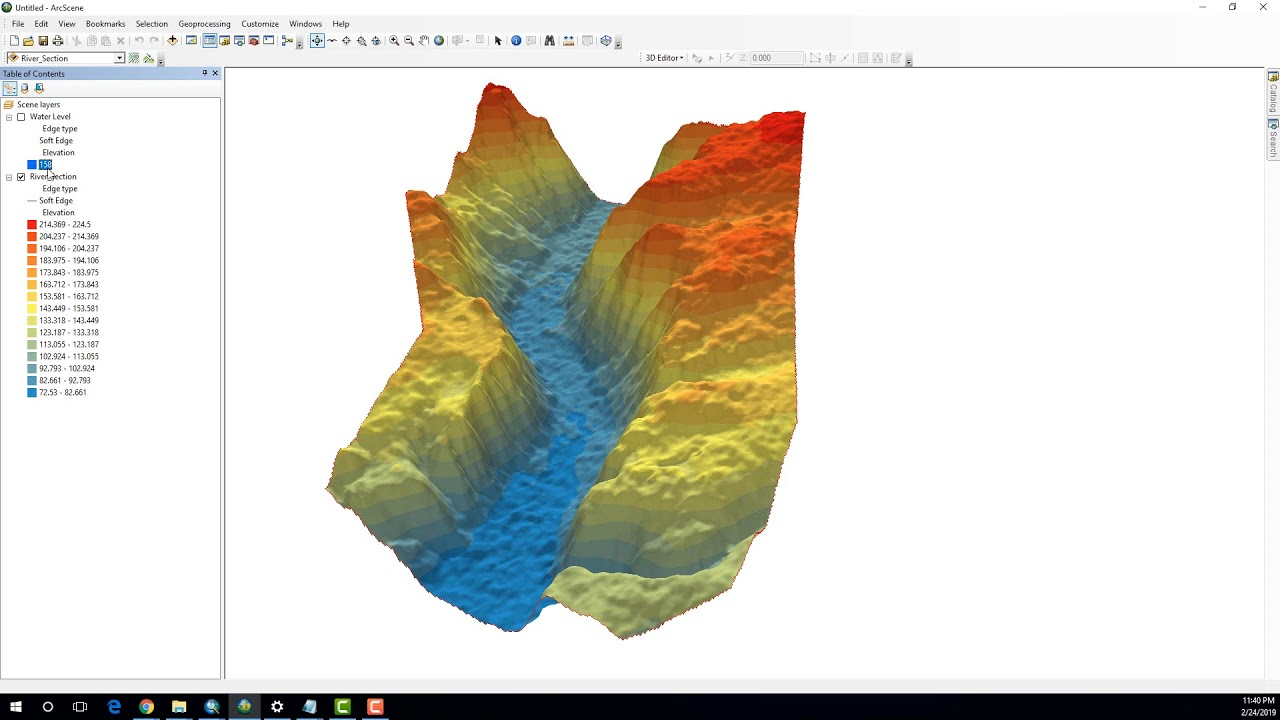# Calculating the Volume of a Raster using 3D Analyst of ArcGIS (ArcMap + ArcScene)Follow GeoDelta Labs on Facebook - https://www.facebook.com/geodeltalabs/This tutorial demonstrates to you how to calculate the volume of a specified river c...

This tutorial demonstrates to you how to calculate the volume of a specified river cross section using the ArcGIS 3D Analyst tool.

## Create Thiessen Polygons using ArcMap and Calculating Thiessen Weights

This tutorial emphasizes on how to create thiessen polygons using ArcMap. Furthermore, in the second half of the tutorial you will learn how to calculate the thiessen weights using a simple 'clip' operation and using the field calculator of ArcGIS

## Flood Animation using 3D Analyst tools of ArcGIS (ArcScene + ArcMap)

This is a cool tutorial showing you how to do a flood inundation visualization using the 3D Analyst tools of ArcScene and ArcMap. However, it should be noted that an accurate flood inundation simulation should always be done using proper hydrodynamic tools such as HEC-RAS, MIKE 11 + MIKE 21 etc.

## Changing the cell size of a Raster (Resampling) using ArcGIS

This tutorial shows you how to increase the pixel size of a raster using ArcGIS. The tool used in ArcMap for this purpose is called Resample

## How to Calculate the Geometry of Shapefiles using ArcMap (with Real Examples)

In this tutorial, I have selected an area in Singapore to calculate few geometrical properties of shapefiles. By the end of this tutorial, you will know how to calculate the area and the perimeter of a polygon, the length of a line and how to calculate the X, Y coordinates of the midpoint of a line using ArcMap tools.

## Learn about the ArcGIS API for JavaScript: start web development

Join us for an overview on developing with the ArcGIS API for JavaScript. This session will cover the basics of setting up your development environment, fundamental debugging techniques, and tips for using the online SDK. Learn about patterns and practices for integration with ArcGIS Online, ArcGIS Server, working with data and widgets.